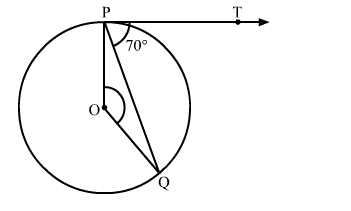# If PT is a tangent to a circle with centre O and PQ is a chord of the circle such that ∠QPT = 70`
Question:

If PT is a tangent to a circle with centre O and PQ is a chord of the circle such that ∠QPT = 70 then find the measure of  ∠POQSolution:

We know that the radius and tangent are perperpendular at their point of contact.
∴∠OPT = 90
Now, ∠OPQ = ∠OPT − ∠TPQ = 90 − 70 = 20
Since, OP = OQ as both are radius
∴∠OPQ = ∠OQP = 20           (Angles opposite to equal sides are equal)
Now, In isosceles △POQ
∠POQ + ∠OPQ + ∠OQP = 180       (Angle sum property of a triangle)
⇒ ∠POQ = 180 − 20 − 20 = 140